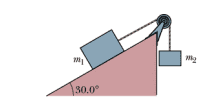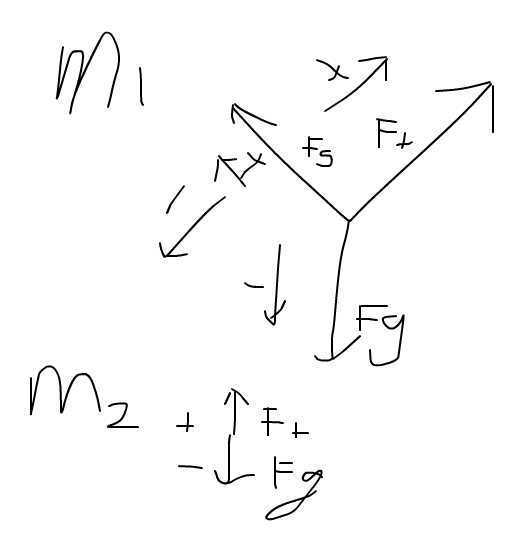# Two blocks on a plane with a pulley

## Homework Statement

A block of mass m1 = 3.70 kg on a frictionless inclined plane of angle 30.0° is connected by a cord over a massless, frictionless pulley to a second block of mass m2= 2.30 kg hanging vertically. What are (a) the magnitude of the acceleration of each block and (b) the direction of the acceleration of m2? (c) What is the tension in the cord?F=ma

## The Attempt at a SolutionI set the coordinate system according to the diagram above.

System: m1
Ft+Fg=m1a
Ft-m1gsinθ=m1a

System: m2
Ft+Fg=m2a
Ft-m2g=m2a
Ft=m2g+m2a

Then I plug in both of them.
m2g+m2a+m1gsinθ=m1a
m2a-m1a=-m1gsinθ-m2g
Then I sub in the values
a=3.15?

I dont know what I did wrong. The correct answer is 0.735.

#### Attachments

•stockzahn
Homework Helper
Gold Member
You've mad a mistake with the signs. Consider the cord as your coordinate and define its positive direction from m1 to m2. Now redo your force balances with all forces in each direction with the same sign.

Last edited:
You've mad a mistake with the signs. Consider the cord as your coordinate and define its positive direction from m1 to m2. Now redo your force balances with all forces in each direction with the same sign.

Why are all forces positive? That doesnt sound right.

stockzahn
Homework Helper
Gold Member
Why are all forces positive? That doesnt sound right.

Sorry, maybe I didn't express myself clearly. Of course you have to distinguish the direction of the forces by using the correct signs. After defining the positive direction, you know all forces pointing in this direction have positive signs and the rest has negative signs.

Sorry, maybe I didn't express myself clearly. Of course you have to distinguish the direction of the forces by using the correct signs. After defining the positive direction, you know all forces pointing in this direction have positive signs and the rest has negative signs.

So how would you define it? Which part of my signs is wrong?

stockzahn
Homework Helper
Gold Member
It's up to you, but as already proposed in my previous post: m1 → m2: positive

It's up to you, but as already proposed in my previous post: m1 → m2: positive

So if to the right of M1, which is on the x axis to the right was positive then the M2 going up would be positive too?

stockzahn
Homework Helper
Gold Member
So if to the right of M1, which is on the x axis to the right was positive then the M2 going up would be positive too?

If your coordinate s along the chord is positive from m1 → m2, then for

m1: sgn(Ft) = + 1 & sgn(Fg) = - 1 ... according to the x-axis you defined
m2: sgn(Ft) = - 1 & sgn(Fg) = + 1 ... as s is positive along the chord in direction m1 → m2

If your coordinate s along the chord is positive from m1 → m2, then for

m1: sgn(Ft) = + 1 & sgn(Fg) = - 1 ... according to the x-axis you defined
m2: sgn(Ft) = - 1 & sgn(Fg) = + 1 ... as s is positive along the chord in direction m1 → m2

Why is Fg positive for m2 Fg? Can you draw it out on the whiteboard which sign should be where?

stockzahn
Homework Helper
Gold Member
Sorry, whiteboard doesn't work on my computer. To see it just draw an arrow parallel to the chord direction pointing from m1 to the pulley and a second one after the pulley pointing in direction of m2. These are the positive directions of your cordinate s. Now compare the direction of each force at m1 with the first arrow: If the force points in the same direction the sign is +. Same for m2 and the second arrow.

Last edited:
haruspex